# Google Code Jam Archive — Round 2 2020 problems

## Overview

Round 2 began with our second pancake problem of 2020: Incremental House of Pancakes. Unlike in Round 1C, you didn't need to do any slicing, but this was tough for a first problem in Round 2, and careful coding was needed. Next came Security Update, which required you to think through the details of an ordering algorithm on a graph. The last two problems were even tougher! Wormhole in One asked you to play some space golf in which the ball could travel between connected holes. Emacs++ was another problem in our series of riffs on programming languages and editors. We just might create a Vim++ or C++++ problem eventually...

Our first fully correct solution to a problem didn't come in until just after the 10 minute mark, and it took around an hour to see our first full solve of every problem except Emacs++. Shortly before the two-hour mark, we got our first scores of 77, missing only Test Set 2 of Emacs++. The only perfect score came in within the last five minutes in the contest, from Benq, who won the round! The next four, all with 77 points, were imeimi, sevenkplus, bmerry, and scottwu. 22 contestants solved at least one test set of the fearsome Emacs++, and Benq and CCCiq were the only contestants to fully solve it.

Over 4000 of our 4500 qualified contestants participated in the round! As usual, please bear with us as we review the results, but the top 1000 scorers in this round win this year's Code Jam T-shirt and advance to Round 3! Advancers have three weeks to practice... but even if you didn't advance, please remember that competing in Round 2 is itself a noteworthy accomplishment. We'll also note that there's a Kick Start round coming up very soon!

Cast

Incremental House of Pancakes: Written and prepared by Pablo Heiber.

Security Update: Written by Pablo Heiber. Prepared by John Dethridge and Kevin Gu.

Wormhole in One: Written by Pablo Heiber. Prepared by Artem Iglikov.

Emacs++: Written by Max Ward. Prepared by Darcy Best and Max Ward.

Solutions and other problem preparation and review by Mohamed Yosri Ahmed, Liang Bai, Darcy Best, Timothy Buzzelli, John Dethridge, Evan Dorundo, Kevin Gu, Jonathan Irvin Gunawan, Md Mahbubul Hasan, Andy Huang, Artem Iglikov, Nafis Sadique, Pi-Hsun Shih, Sudarsan Srinivasan, Ian Tullis, and Max Ward.

Analysis authors:

• Incremental House of Pancakes: Pablo Heiber.
• Security Update: Pablo Heiber.
• Wormhole in One: Artem Iglikov.
• Emacs++: Darcy Best and Max Ward.

## A. Incremental House of Pancakes

### Problem

Every morning at The Incremental House of Pancakes, the kitchen staff prepares all of its pancakes for the day and arranges them into two stacks. Initially, the stack on the left has L pancakes, and the stack on the right has R pancakes.

This restaurant's customers behave very consistently: the i-th customer to arrive (counting starting from 1) always orders i pancakes. When the i-th customer places their order of i pancakes, you take i pancakes from the stack that has the most pancakes remaining (or from the left stack if both have the same amount). If neither stack has at least i pancakes, the restaurant closes and the i-th customer does not get served any pancakes. You never complete an order using pancakes from both stacks.

Given the initial numbers of pancakes in each stack, you want to know how many customers will be served, and how many pancakes will remain in each stack when the restaurant closes.

### Input

The first line of the input gives the number of test cases, T. T test cases follow. Each test case consists of a single line containing two integers L and R: the initial numbers of pancakes in the left and right stacks, respectively, as described above.

### Output

For each test case, output one line containing `Case #x: n l r`, where `x` is the test case number (starting from 1), `n` is the number of customers who will be served, and `l` and `r` are the numbers of pancakes that will remain in the left and right stacks, respectively, when the restaurant closes.

### Limits

Time limit: 20 seconds per test set.
Memory limit: 1GB.
1 ≤ T ≤ 1000.

1 ≤ L ≤ 1000.
1 ≤ R ≤ 1000.

1 ≤ L ≤ 1018.
1 ≤ R ≤ 1018.

### Sample

Sample Input
```3
1 2
2 2
8 11
```
Sample Output
```Case #1: 1 1 1
Case #2: 2 1 0
Case #3: 5 0 4
```

In Sample Case #1, the first customer gets 1 pancake from the right stack, leaving 1 pancake in each stack. The second customer wants 2 pancakes, but neither stack has enough for them, even though there are 2 pancakes in total.

In Sample Case #2, the first customer gets 1 pancake from the left stack, because both stacks have the same amount. This leaves 1 pancake in the left stack and 2 in the right stack. The second customer wants 2 pancakes, which you serve to them from the right stack, emptying it. When the third customer arrives, neither stack has 3 pancakes, so no more orders are fulfilled.

In Sample Case #3, the first customer is served from the right stack, leaving 8 pancakes in the left stack and 10 in the right stack. The second customer is also served from the right stack, leaving 8 pancakes in each stack. The third customer is served from the left stack, leaving 5 pancakes there and 8 in the right stack. The fourth customer is then served from the right stack, leaving 4 pancakes there. Serving the fifth customer empties the left stack, and then there are not enough pancakes remaining in either stack to serve a sixth customer.

## B. Security Update

### Problem

The Apricot Rules company just installed a critical security update on its network. The network has one source computer, and all other computers in the network are connected to the source computer via a sequence of one or more direct bidirectional connections.

This kind of update propagates itself: once a computer receives the update for the first time, that computer immediately begins to transmit the update to all of the computers that are directly connected to it. Each of the direct connections has a latency value: the number of seconds needed for that connection to transmit the update (which is the same in either direction). Therefore, the update does not spread to all computers instantly.

The Apricot Rules engineers do not know any of these latency values, but they know that they are all positive integers. They would like your help in figuring out what these latency values could be, based on how they saw the update spread in a recent experiment.

The Apricot Rules engineers installed the update only on the source computer and then waited for it to propagate throughout the system until every computer was updated. They recorded some information about how the update spread. Specifically, for every computer K other than the source computer, you know exactly one of two things.

• The exact time in seconds between the time when the source computer received the update and the time when K first received the update.
• The number of other computers (including the source computer) that first got the update strictly before K.

Notice that multiple computers may have received the update at the exact same time.

You are required to compute a latency in seconds for each of the direct connections between two computers. Each latency value must be a positive integer no greater than 106. The set of latencies that you provide must be consistent with all of the known information. It is guaranteed that there is at least one consistent way to assign latencies.

### Input

The first line of the input gives the number of test cases, T. T test cases follow. Each case begins with one line containing two integers C and D: the number of computers and the number of direct connections, respectively. The computers are numbered from 1 to C, with computer 1 being the source computer.

The next line contains C-1 integers X2, X3, ..., XC. A positive Xi value indicates that computer i received the update Xi seconds after computer 1. A negative Xi value indicates that -Xi other computers received the update strictly before computer i; this value includes the source computer.

After that, there are D more lines that represent the D direct connections in the network. The i-th of these lines contains two integers Ui and Vi, indicating that computers Ui and Vi are directly connected to each other.

### Output

For each test case, output one line containing ```Case #x: y1 y2 ... yD```, where `x` is the test case number (starting from 1) and `yi` is a positive integer not more than 106 representing the latency, in seconds, assigned to the i-th direct connection.

### Limits

Time limit: 20 seconds per test set.
Memory limit: 1GB.
1 ≤ T ≤ 100.
2 ≤ C ≤ 100.
C - 1 ≤ D ≤ 1000.
1 ≤ Ui < ViC, for all i.
(Ui, Vi) ≠ (Uj, Vj), for all i ≠ j.
All computers (except the source computer) are connected to the source computer through a sequence of one or more direct connections.
There exists at least one way of assigning latency values that is consistent with the input.

#### Test Set 1 (Visible Verdict)

-C < Xi < 0, for all i. (You get the second type of information for all computers.)

#### Test Set 2 (Hidden Verdict)

-C < Xi ≤ 1000, for all i.
Xi ≠ 0, for all i.

### Sample

Sample Input
```3
4 4
-1 -3 -2
1 2
1 3
2 4
3 4
4 4
-1 -1 -1
1 4
1 2
1 3
2 3
3 2
-2 -1
2 3
1 3
```
Sample Output
```Case #1: 5 10 1 5
Case #2: 2020 2020 2020 2020
Case #3: 1000000 1000000
```

In Sample Case #1, the following picture represents the computer network that is illustrated by the sample output. The i-th computer is represented by the circle with the label i. A line linking two circles represents a direct connection. The number on each line represents the latency of the direct connection.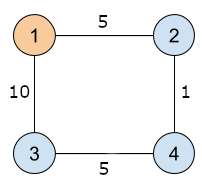In Sample Case #2, the first three connections need to have the same latency, while the fourth can have any valid latency. Note that -2, 0, 1000001, and 3.14 are examples of invalid latencies.

In Sample Case #3, remember that the connections are bidirectional, and so the update can travel from computer 3 to computer 2. Any two valid latency values work here.

The following case could not appear in Test Set 1, but could appear in Test Set 2:

```1
6 9
10 -2 -5 15 20
1 2
1 3
2 3
2 4
2 5
3 5
3 6
4 5
5 6
```

One of the correct outputs is `10 12 4 15 8 3 9 7 5`, as illustrated by the picture below.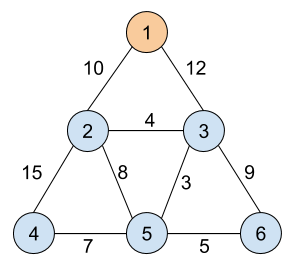## C. Wormhole in One

### Problem

You are participating in an inter-galactic hyperspace golf competition, and you have advanced to the final round! You are really determined to triumph, and so you want to prepare a winning strategy.

In hyperspace golf, just as in conventional golf, you hit a ball with a club, which sends the ball in a direction chosen by you. The playing field in hyperspace golf is a 2-dimensional plane with points representing the different holes. The ball is also represented by a point, and you get to choose where the ball starts, as long as it is not in the same place as a hole.

Since this is hyperspace golf, the players are allowed to turn some pairs of holes into wormholes by linking them together. Each hole can be either left as a normal hole, or linked to at most one other hole (never to itself). Wormholes are undirected links, and can be traversed in either direction.

Because the environment is frictionless, when you hit the ball, it moves in a straight direction that it maintains forever unless it reaches a hole; call that hole h. Upon touching hole h, the ball stops if h is not connected to another hole. If h is connected to another hole h', then the ball immediately comes out of h' and continues moving in the same direction as before.

You know the location of each hole. You want to maximize the number of distinct holes you can touch with a single hit. With that goal in mind, you want to pick the ball's starting location, the direction in which to send the ball, and which pairs of holes, if any, to link together as wormholes. The ball cannot start in the same place as a wormhole. When the ball goes through a wormhole, both the hole it goes into and the hole it comes out of are counted towards your total. Each hole is only counted once, even if the ball goes into it or comes out of it (or both) multiple times. If the ball stops in a hole, that hole also counts toward your total.

### Input

The first line of the input gives the number of test cases, T. T test cases follow. Each case begins with one line containing a single integer N: the total number of holes. The following N lines contain two integers each: Xi and Yi, representing the X and Y coordinates, respectively, of the i-th hole.

### Output

For each test case, output one line containing Case #x: y, where x is the test case number (starting from 1) and y is the maximum number of distinct holes you can touch if you make optimal decisions as described above.

### Limits

Time limit: 30 seconds per test set.
Memory limit: 1GB.
1 ≤ T ≤ 100.
-109Xi ≤ 109, for all i.
-109Yi ≤ 109, for all i.
(Xi, Yi) ≠ (Xj, Yj), for all i ≠ j. (No two holes are at the same coordinates.)

1 ≤ N ≤ 7.

1 ≤ N ≤ 100.

### Sample

Sample Input
```5
2
0 0
5 5
3
0 0
5 5
5 0
5
0 0
5 5
5 0
3 2
2 4
7
0 0
1 1
2 1
3 1
8 2
11 2
14 2
1
-1000000000 1000000000
```
Sample Output
```Case #1: 2
Case #2: 3
Case #3: 4
Case #4: 7
Case #5: 1
```

In Sample Case #1, we can connect the two holes with a wormhole so that we could touch both of them by sending the ball into either one. Notice that without the wormhole, the ball would just stay in the first hole it touches, so it would be impossible to touch more than one hole.In Sample Case #2, we can connect the holes at (0, 0) and at (5, 5). We can then hit the ball from position (4.9, 5), for example, in the positive horizontal direction so that it first touches the hole at (5, 5). It goes into that hole and comes out of the hole at (0, 0), retaining its positive horizontal direction of movement. Finally, it touches the hole at (5, 0), and stops (since there is no wormhole linked to that hole).In Sample Case #3, we can connect the pair of holes at positions (0, 0) and (5, 0), and also the pair of holes at positions (3, 2) and (5, 5). Hitting the ball from (4, -1) towards the hole at (5, 0) makes it touch the holes at positions (5, 0), (0, 0), (5, 5) and (3, 2), in that order.In Sample Case #4, we can connect the pairs of holes at positions (0, 0) and (1, 1), the pair of holes at positions (2, 1) and (11, 2), and also the pair of holes at positions (8, 2) and (14, 2). Hitting the ball from (-1, 0) towards the hole at (0, 0) makes it touch the holes at the following positions, in this order: (0, 0), (1, 1), (2, 1), (11, 2), (14, 2), (8, 2), (11, 2), (2, 1), and (3, 1). Note that although the holes at positions (11, 2) and (2, 1) are touched twice, they are only counted once each for the answer, since the problem asks for a count of distinct holes.In Sample Case #5, there is only one hole, and we can hit the ball into it without needing to consider wormholes at all. (For what it's worth, we can choose any starting location we want, even outside of the allowable range of coordinates for holes.)## D. Emacs++

### Problem

In 2016's Distributed Code Jam, we introduced the Lisp++ language for Lisp fans who prefer a higher density of parentheses. Here is a reminder of how the language's syntax works:

A Lisp++ program is a string of balanced parentheses. More formally, a Lisp++ program consists of one of the following. (In this specification, C stands for some program code — not necessarily the same code each time.)

• `()` Literally, just an opening parenthesis and a closing parenthesis. We say that this `(` matches this `)`, and vice versa.
• `(`C`)` A program within a pair of enclosing parentheses. We say that this `(` matches this `)`, and vice versa.
• CC Two programs (not necessarily the same), back to back.

This year, we are pleased to announce Emacs++, a text viewer for Lisp++. Emacs++ displays a Lisp++ program of length K as a single long line with a cursor that you can move around. The cursor is a "block cursor" that is always located on one of the K characters in the program, rather than between characters.

At any point, you can perform one of the following three actions to move the cursor. (i represents the current position of the cursor, counting starting from 1 for the leftmost position.)

• Move the cursor one character to the left (or, if the cursor is already on the leftmost character, does nothing). This takes Li seconds.
• Move the cursor one character to the right (or, if the cursor is already on the rightmost character, does nothing). This takes Ri seconds.
• Teleport the cursor to the parenthesis matching (as described above) the parenthesis that is the i-th character. This takes Pi seconds.

We think Emacs++ will be simple for power users, but we still need to understand how efficient it is. We have a single Lisp++ program and list of Q queries about that program; each query consists of a start position Sj and an end position Ej. To answer the j-th query, you must determine the smallest possible amount of time Nj (in seconds) that it will take to take the cursor from position Sj to position Ej, if you make optimal decisions.

Please output the sum of all of those Nj values.

### Input

The first line of the input gives the number of test cases, T. T test cases follow. The first line of a test case contains two integers K, which is the length of the Lisp++ program, and Q, which is the number of queries.

The second line of a test case contains a string P of K characters, each of which is either `(` or `)`, representing a Lisp++ program (string of balanced parentheses), as described above.

The third, fourth, and fifth lines of a test case each contain K integers. The i-th integers in these lines are the values Li, Ri, and Pi, respectively, that are described above.

The sixth and seventh lines of a test case each contain Q integers. The j-th integers in these lines are Sj and Ej, respectively, that are described above.

### Output

For each test case, output one line containing `Case #x: y`, where `x` is the test case number (starting from 1) and `y` is the sum of the Nj values that are described above.

### Limits

Time limit: 60 seconds per test set.
Memory limit: 1GB.
1 ≤ T ≤ 100.
K = 105 and Q = 105, for at most 9 test cases.
2 ≤ K ≤ 1000 and 1 ≤ Q ≤ 1000, in all other cases.
length of P = K P is a string of balanced parentheses, as described above.
1 ≤ SjK, for all j.
1 ≤ EjK, for all j.

#### Test Set 1 (Visible Verdict)

Li = 1, for all i.
Ri = 1, for all i.
Pi = 1, for all i.

#### Test Set 2 (Hidden Verdict)

1 ≤ Li ≤ 106, for all i.
1 ≤ Ri ≤ 106, for all i.
1 ≤ Pi ≤ 106, for all i.

### Sample

Sample Input
```1
12 5
(()(((()))))
1 1 1 1 1 1 1 1 1 1 1 1
1 1 1 1 1 1 1 1 1 1 1 1
1 1 1 1 1 1 1 1 1 1 1 1
7 4 4 12 5
12 11 10 1 6
```
Sample Output
```Case #1: 10
```

In the sample case, which obeys the limits for Test Set 1, all of the time costs are the same (1 second per move).
The shortest times for the queries are as follows:

1. Move right from 7 five times to 12 taking 5 seconds.
2. Teleport from 4 to 11 taking 1 second.
3. Teleport from 4 to 11, then move left to 10 taking 2 seconds.
4. Teleport from 12 to 1, taking 1 second.
5. Move right from 5 to 6 taking 1 second.
Thus, the sum of query times is 5+1+2+1+1 = 10 seconds.

## Analysis — A. Incremental House of Pancakes

### Test Set 1

Test Set 1 is small enough that we can simulate the process. Since each step removes at least one pancake from a stack, this takes at most L + R operations. One thing we can notice is that because the i-th step removes i pancakes, the first i steps together remove i × (i + 1) / 2 pancakes. This means that the number of steps is actually bounded by 2 × sqrt(L + R), which means the simulation algorithm is also O(sqrt(L + R)). It would work for limits that are much larger than the ones in this test set!

### Test Set 2

Unfortunately, O(sqrt(L + R)) is still too slow for the 1018 limits in Test Set 2, especially with 1000 cases to go through!

We can simulate multiple steps at a time by noticing that there are two distinct phases of the process. The first phase uses a single stack: the one that starts out with more pancakes. The second phase begins when that stack has a number of pancakes remaining that is less than or equal to the number in the other stack. Notice that if the left stack is the one we serve from in phase 1, it is possible that it is also the first one to be used in phase 2. It is also possible that no customer is served in either phase.

We may serve a lot of customers in phase 1 depending on the difference in size between the stacks at the beginning. If we serve i pancakes from one stack, we remove i × (i + 1) / 2 pancakes from it, so we can efficiently calculate how many customers we can serve in phase 1 by finding the largest i1 such that i1 × (i1 + 1) / 2 is less than or equal to the difference in number of pancakes between the two stacks at opening time. We can calculate that either by solving a quadratic equation and rounding carefully, or by using binary search.

The second phase is where the magic happens. Let us say that when serving customer i, stack X is used and Y is not, but then we serve customer i + 1 from stack Y. Therefore, stack X lost i pancakes and stack Y lost i + 1 pancakes. Since X was used instead of Y for customer i, X must have had no fewer pancakes than Y had at that time. Since X lost fewer pancakes than Y, X must have had more pancakes than Y after we served customers i and i + 1. This means if we ever use two different stacks in the order (X, Y), we must use X next. And, using the same reasoning with the roles reversed, we have now have used (Y, X) most recently, so we will use Y next, and so on. So, once we have used both piles, we always go on alternating between them.

We can use this observation to efficiently determine what happens in phase 2. After updating the original totals by subtracting the pancakes served in phase 1, we know which stack is used first in phase 2. The first stack will be used to serve customers i1 + 1, i1 + 3, i1 + 5, ... which means that if it is used for c1 customers, a total of (i1 × c1) + (c1)2 pancakes are served from it. At this point, we know the value of i1, so once again, we can calculate c1 by solving a quadratic equation or binary search. The other stack is similar, since it will be used to serve customers i1 + 2, i1 + 4, i1 + 6, ... so if it is used for c2 customers, a total of ((i1 + 1) × c2) + (c2)2 pancakes will be served from it. So the final number of customers served is i1 + c1 + c2. The total numbers of pancakes served from each stack come from the quantities in phase 2, with the quantity from phase 1 added to whichever stack was first.

If we use binary searches, each phase requires O(log(L + R)) time. If we directly solve the quadratic equations, each phase is actually constant time. Either is fast enough for the limits of this problem.

Notice that solving quadratic equations may be harder than usual, since typically that involves computing some square roots. While most languages provide a way to do that, they do it with double precision floating point, which does not have enough precision for this problem and can lead to off-by-one errors. We should either compute square roots directly on integers (by binary searching for the answer, for example) or use the built-in function, and then check the returned value and other values in its vicinity to find the correct rounded result.

Test Data
info We recommend that you practice debugging solutions without looking at the test data.

## Analysis — B. Security Update

Let Ri be the number of computers that receive the update before computer i, and Ti be the time between computer 1 and computer i receiving the update. For each i, the input gives us exactly one of these numbers. We can set R1 = T1 = 0 for convenience.

### A simplified problem

Let us assume for now that we have all the Tis. If computers i and j share a direct connection and Ti=Tj, then any path that gets to computer i in time Ti does not go through computer j, and vice versa, because all latencies are positive. Therefore, we can assign any positive latency to all those connections. If computer i has a given Ti, it means that any connection coming from computer j with Tj < Ti needs to have a latency of at least Ti-Tj, or otherwise the update could get to computer i in less than Ti time by getting to computer j in Tj time and then using that connection. In addition, for at least one j, the latency of the connection between i and j has to be exactly Ti-Tj, or otherwise the time for the update to reach computer i would be larger than Ti. One simple way to solve this problem is to make all connections between computers with different T values have a latency of exactly |Ti-Tj|; this takes O(D) time.

Notice that the algorithm above finds a valid assignment for any set of Tis. To solve the actual test sets, we are left with the problem: given some Tis and some other Ris, assign all of the non-given values in a way such that sorting the computers by Ti leaves them sorted by Ri, and vice versa. In particular, computers with equal T values should have equal R values, and vice versa.

### Test Set 1

In this test set, we can solve the subproblem from the previous section by setting Ti := Ri.

### Test Set 2

For Test Set 2, we again focus on solving the subproblem. We do that by first ordering the computers by what is going to be their final Ti value (or equivalently, by their final Ri value). We can partition the set of computers other than the source computer into two: those for which we know Ri (part R) and those for which we know Ti (part T). We can sort each of those in non-decreasing order of the known value. We now have 2 sets that are in the right relative order, and need to merge them as in the last step of Merge Sort. We assign the source computer first. Then we iterate through the remaining C-1 slots in order. Suppose we have already merged N computers, and let computer k be the last one of those. Let i and j be the first computers remaining in parts R and T, respectively. If Ri ≤ N, we take computer i next and assign Ti := Tk if Ri = Rk, and Ti := Tk+1 otherwise. If Ri > N, we take computer j next and assign Rj := Rk if Tj = Tk and Rj := N otherwise.

We can prove that if the original set of values is consistent with at least one latency assignment (which the statement guarantees), this generates a valid order and assignment of missing values, and moreover, it generates one in which the T value of the last computer in the order is minimal. We do that by induction on the number of computers. For a single computer, this is trivially true. Suppose we have C > 1 computers. By our inductive hypothesis, the first C-1 computers in the order were ordered and assigned values in a consistent way, with a minimal T value for the last computer among all options. Let us say the last computer in the full order is computer i, and the next-to-last computer is computer j. By definition of how we assign missing values, Ri = Rj if and only if Ti = Tj. If indeed Ri = Rj and Ti = Tj, then the condition for the final assignment is equivalent to the inductive hypothesis. If computers i and j come from the same part, then the ordering choice between them was fixed, and the assignment of T values if needed is clearly minimal. So consider further the case in which computer i comes from a different part than computer j, and their R and T values are different. We have two cases: either computer i was in part R, or in part T.

If computer i was in part R, then its assigned T value is by definition the largest among all computers, and it's the smallest possible for it to go after computer j, whose value is minimal by the inductive hypothesis. As for the order, RiC-1 per the limits. Since computer j comes from part T and was chosen for position C-1 (when N was C - 2), that means Ri > C - 2. Therefore, Ri=C-1, and the chosen position is correct.

If, on the other hand, computer i was in part T, then its T value is minimal because Ti is fixed. As for the order, notice that all computers have either a T value strictly less than Ti or an R value strictly less than C-1, so none of them could have been last. By the inductive hypothesis, Tj is minimal among all possible orders, which means, by the existence of a full assignment, it has to be Tj < Ti, which implies the consistency of the final order and value assignment.

Test Data
info We recommend that you practice debugging solutions without looking at the test data.

## Analysis — C. Wormhole in One

### Test Set 1

As the limits for Test Set 1 are very small, we should be able to apply a brute-force algorithm and check all possible directions, starting points, and links between holes. However, there are infinitely many directions and starting points. Let's find a way to work with a finite number of possibilities.

First, let us observe that in order for the ball to touch more than two holes, some pairs of holes must be on lines parallel to the chosen direction. Otherwise, the best we can do is to link together two holes; the ball will go through them and will not touch any other holes.

So, when choosing an initial hit direction, we only need to consider those that are parallel to lines that connect pairs of holes.

Also, the exact starting point is not important. We can decide which hole we want to enter first, and then, regardless of which hit direction we choose, we can position the ball such that it will enter that hole (before any others). For example, because the holes are always at unique integer coordinates, we can choose a starting distance of 0.1 from the hole, in the direction that is the opposite of our hit direction.

Now, we can try all possible starting holes and linking schemes — with a recursive backtracking algorithm, for example — and choose the combination that touches the largest number of holes. This should be enough to pass Test Set 1.

### Test Set 2

Suppose for now that we have chosen the direction of the hit. Let's calculate the maximum possible answer given that decision.

Imagine lines parallel to the chosen direction and going through all of the holes. Notice that each hole is on at most one such line. We will call a line an odd line if it contains an odd number of holes (but more than one), and an even line if it contains an even number of holes (at least two). If there are lines which only contain one hole, we call these holes standalone holes.

Let's also consider the holes along each line to be ordered in the chosen direction.

Note that:

• We cannot touch more than two standalone holes: one at the beginning, and one at the very end of the ball's journey.
• In the best case, we would touch all non-standalone holes.

Let's say we have Codd total holes on the odd lines, Ceven total holes on the even lines, and C1 standalone holes. Then the answer is not greater than Codd + Ceven + min(2, C1).

To touch two standalone holes, we should touch an even number of holes between them. To understand why, note that first of the standalone holes must be an entry to a wormhole, and the second one must be an exit from another wormhole. All of the holes that the ball touches in between those starting and ending holes must be linked in pairs by wormholes, which means there should be an even number of holes. This also means that if the number of non-standalone holes is odd, then we will not be able to touch two standalone holes; in such a case, the answer will not be greater than Codd + Ceven + min(1, C1).

As we will see, these upper limits can actually be achieved, so the answer is:

• Codd + Ceven + min(1, C1), if Codd + Ceven is odd
• Codd + Ceven + min(2, C1), if Codd + Ceven is even

Note that the parity of Codd + Ceven is the same as the parity of Codd, as Ceven is always even.

Let's construct the linking scheme for the case when Codd is even and C1 is greater than 1:

1. Connect one standalone hole to the first hole of any even line.
2. Connect the other holes on that line pairwise consecutively (the last hole will remain unconnected).
3. Connect the last hole on that line to the first hole of another even line, and repeat steps 2 and 3 until only odd lines are left untouched.
4. Connect the last hole of the last even line to the first hole of any odd line.
5. Connect the second hole of that odd line (A) to the second hole of another odd line (B).
6. Connect the last hole of line B to the first hole of line B.
7. Connect the last hole of line A to the first hole of another odd line.
8. Connect the remaining holes of line A in consecutive pairs.
9. Connect the remaining holes of line B in consecutive pairs.
10. Repeat steps 5-9 until all the odd lines are used. When there are no more odd lines, connect the last hole of the last odd line to an unused standalone hole.

This scheme can be easily modified for the other cases, when Codd is odd, and/or C1 is less than 2.

Summarizing this, we can make full use of all of the odd and even lines and up to two standalone holes.

To calculate the number of holes on each line for a given direction, we can iterate through all ordered pairs of the holes and find the equation of the line that connects them in the form y = mx + y0. Now, for each m, we store how many times we see each y0. This number will be equal to the number of pairs of holes on this line, which we can use to calculate the number of holes. Note that we only need to do this once, as we will get the counts for all directions we are interested in.

Now, we can iterate through all the directions and calculate the answer for each one as described earlier by iterating through all the lines parallel to the current direction. Our final answer will be the maximum over the answers for all directions.

Note that even though there are O(N2) directions, and O(N) lines parallel to a direction, the total number of lines for all directions is O(N2), as every line can be parallel to two (opposite) directions. So the total time complexity of this solution implemented optimally is O(N2), but slower implementations might also pass.

Test Data
info We recommend that you practice debugging solutions without looking at the test data.

## Analysis — D. Emacs++

### Test Set 1

TL;DR: Think of the brackets like a tree where a position's parent is the closest pair of brackets that contain that position. Go to the Lowest Common Ancestor, then back down the tree.

With this problem, our first thought may be to write a breadth first search for each query and add up the values. Unfortunately, this is much too slow for the bounds provided. Thankfully, we can make use of the structure of the Lisp++ program and the fact that all movement costs are 1 (going left, going right, or going to the matching bracket—we will call going left or right "walking" and going to the matching bracket "jumping" to make the explanation easier).

Two somewhat simple observations are needed before we can talk about the intended solution. The first observation needed is that we almost always want to jump instead of walking inside of a pair of brackets. If we are at a bracket endpoint, and jumping to the other endpoint doesn't make us "overshoot" the query's destination, then we should take that jump instead of walking there. The second observation needed is that if a pair of brackets contains both our current position and our destination, we should never move outside of that pair of brackets.

Using just these two observations, let's discuss what an optimal path looks like for a specific query. Consider the closest pair of brackets that contains both the start and end of the query: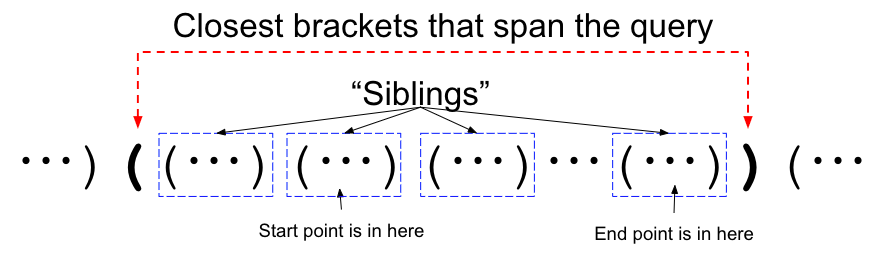The optimal path for this query must move "up" to this level: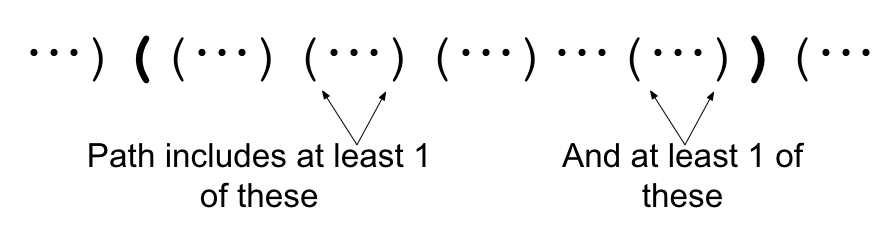One we are at this level, we jump along the top level of the siblings until we are at the brackets for the end query, then move "down" to the query answer. Note that we can jump either left or right (one of which will wrap around when we hit the bracket's endpoint) so we should check both and take the minimum.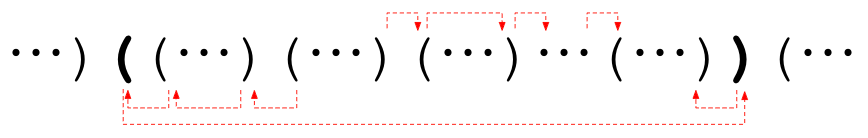At this point, we treat the Lisp++ program as a tree with the parents in the tree being the closest pair of brackets that enclose us. Note that one of the parents is strictly closer than the other, so we will always go to that parent first on our path "up" or "down" the tree. The layer at the top is simply the lowest common ancestor in the tree, which can be computed in O(log K) time.

### Test Set 2

TL;DR: (1) Find two pairs of brackets that partition the Lisp++ program into 4 disjoint sections. (2) Compute the shortest path from the break points to answer queries that go from one region to another. (3) Recurse on the 4 subregions.

For Test Set 2, some of the properties we used are no longer available to us. In particular, it may now be optimal to venture outside of the LCA described above. There are two main classes of solutions that are used to solve this test set. One is a modification of the LCA algorithm above. We will show the other here because it demonstrates an algorithm that is less traditional. Rather than answering the queries one at a time, we will employ a method where we can solve them in batches.

The key property we will be using is the ability to partition the Lisp++ program into multiple (almost) independent sections. First, let's start with a crucial observation. Consider a pair of matching brackets. The only way to get from inside the brackets to outside the brackets is to cross through the brackets themselves. These brackets that are used to split the input into the different sections will be called the special brackets throughout the explanation.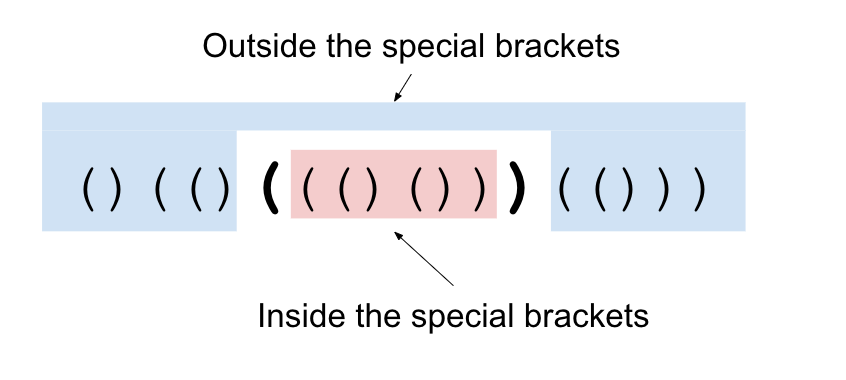We can use this fact to answer queries differently. Rather than computing the distance from the starting point to the ending point of the query, we can compute the distance from each of the special brackets to the query's starting point and from the special brackets to the query's ending point. Since we know that any shortest path must go through one of the special brackets, we know that the sum of these two distances is the answer to the query.

At first, it doesn't seem like this helps us. However, note that we can compute the answer to all queries that go from inside the brackets to outside the brackets at once! Just compute the distance from the special brackets to all locations (using Dijkstra's algorithm, for example) and use the method above.

Now what about all of the queries that start and end inside the special brackets (or outside the special brackets)? Well, the shortest path from the start to the end might go through the special brackets we chose, so we should make note of the potential answer if it does. Now, we're only interested in paths that do not use the special brackets.

Thus, we can split our problem up into two sub-problems: the "inside" part and the "outside" part. Split the queries up into their appropriate parts (inside and outside) and recursively solve each of these. Note that the special brackets can be removed completely since we know the answers to any query involving them. There is one issue, though: this isn't necessarily fast enough. ☹ If we choose our special brackets poorly so that the "inside" is always a short string, then this algorithm will need O(K2) time. In order to make this fast, we need to ensure that both subproblems are about half the size of the original problem. If we add in some more heuristics and choose our bracket pair randomly, we can make our code faster on average, but it is not guaranteed to pass the data. There is a slight tweak we can make described below which will save us!

Instead of spliting the string into 2 sections, we will instead split the string into 4 sections. Consider a specific pair of brackets. Those brackets' parents are the closest pair of brackets that enclose them. If we split using a pair of brackets and their parents' brackets, we split our input into 4 sections. Note that it is impossible to get from those inner side pieces to the other side without crossing one of our two pairs of special brackets (since the parents are the closest brackets to our original brackets that would allow us to do that).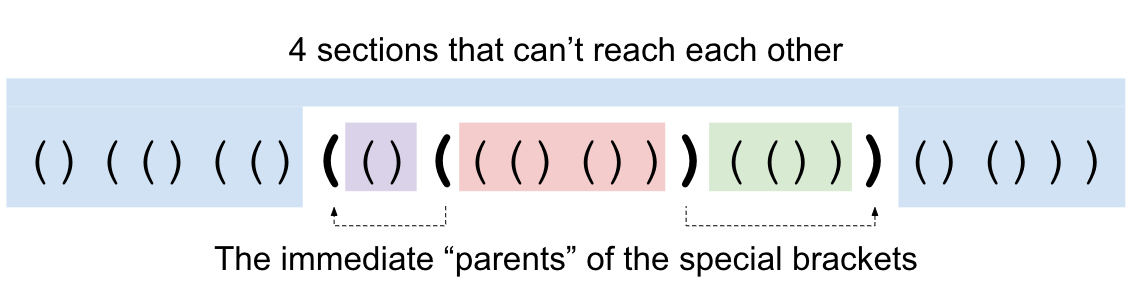This small change looks like we just made things more complicated, but it solves our issue from above! First, let's add a pair of brackets to the outside of our string and set its Li, Ri, and Pi to infinity. This way, all pairs of brackets have a parent, except for this new infinite pair we added.

Let's consider all the bracket pairs whose span (from starting bracket to closing bracket, inclusive) includes the middle bracket (there are two "middle" brackets; we can choose either). Call these brackets the "middle line brackets". The middle line brackets will form a chain in which each bracket pair nests under another middle line bracket, or is the outermost bracket that we added. Our middle line brackets have some nice properties that we can use.

If we consider the middle line brackets from outermost to innermost, we can observe that the spans of the brackets go from containing more than half the characters (the outermost bracket that we added spans the whole string) in our Lisp++ program, to containing at most half of the characters. This is because they are always getting smaller, and the innermost one spans at most half of all characters. Let's consider this "pivot point". It comprises a pair of consecutive middle line brackets in which one spans more than half, and it has a direct child that is also a middle line bracket that spans at most half. If we take these two brackets and cut them out of the string, we will have broken the string into 4 disjoint (possibly empty) parts, none of which contain more than half of the characters in the string.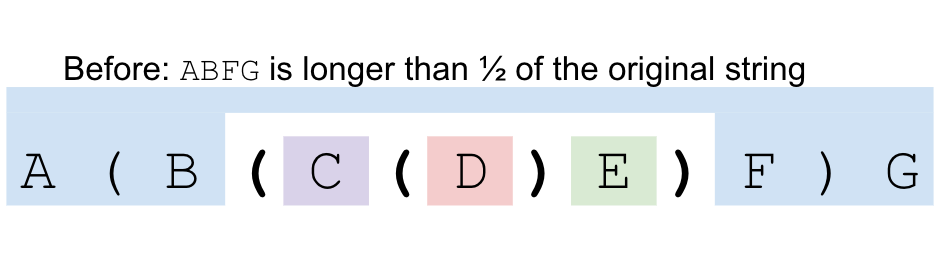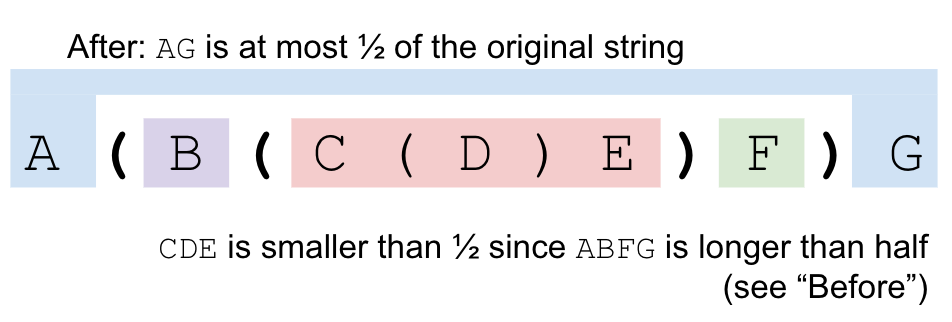Why? Let's say our brackets look like this `A ( B ( C ) D ) A`. We know that since the outer bracket pair spans more than half, the region `A` contains less then half of the characters. We know that the inner bracket either crosses or touches the middle line, so the regions `B` and `D` contain less than half of the characters. Finally, `C` has at most half (remember that we chose `C` specifically because it was the first middle line bracket that contained at most half of the characters). Note that we cannot get from one region to another without crossing a special bracket. In particular, we cannot get between `B` and `D` because the outer special brackets are the parent of the inner special brackets.

Thus, we can solve the problem by finding the two pairs of special brackets we are going to split on, using Dijkstra's algorithm to answer the queries that go between different regions (as well as compute potential answers for those queries that do not), and then recursing into the 4 sub-problems. Since each recursion cuts the length of the input string in half, we recurse at most O(log K) times. The sum of the strings at any particular depth of the recursion is at most the length of the original string. So the total work we needed to do at each layer is at most O(K log K) to run Dijkstra from our 4 special brackets. Also, each query is looked at at most once on each layer of the recursion, so the total complexity is O(K log2 K + Q log K).

### Some Common Issues

Here is a list of common issues that might explain a Wrong Answer or Time Limit Exceeded verdict:

• The edges are directed! This means that the distance from A to B is not necessarily the same as the distance from B to A. For the intended solution, this means we need to run Dijkstra's algorithm twice, not just once.
• If we place values in our code about "infinity", those values must be large enough, but not so large as to cause overflows.
• Picking a random edge and just breaking it into doing inside and outside is in general too slow. Even if we break the left and the right apart if they're not connected, we can run into issues. Consider the following. Let X=`((((( ... )))))`, be of length sqrt(N). If the input is XXXXX ... XXXX sqrt(N) times, then picking randomly is not very efficient. In order to split the input, we need to get lucky and hit one of the outer points of X in order to really cut down on the size of the input.
Test Data
info We recommend that you practice debugging solutions without looking at the test data.

## Statistics — D. Emacs++

### Test set 1: 22 correct solutions (0.5% solve rate)

First
imeimi C++, 111:33
CCCiq C++, 113:21
sevenkplus C++, 118:00
bmerry C++, 126:54
kuroni C++, 127:36
Shortest
Itst C++, 3004 bytes
kuroni C++, 3396 bytes
gs18115 C++, 3512 bytes
sevenkplus C++, 3688 bytes
pashka C++, 4314 bytes

### Test set 2: 2 correct solutions (0.0% solve rate)

First
CCCiq C++, 116:48
Benq C++, 145:13
Shortest
CCCiq C++, 7157 bytes
Benq C++, 11270 bytes